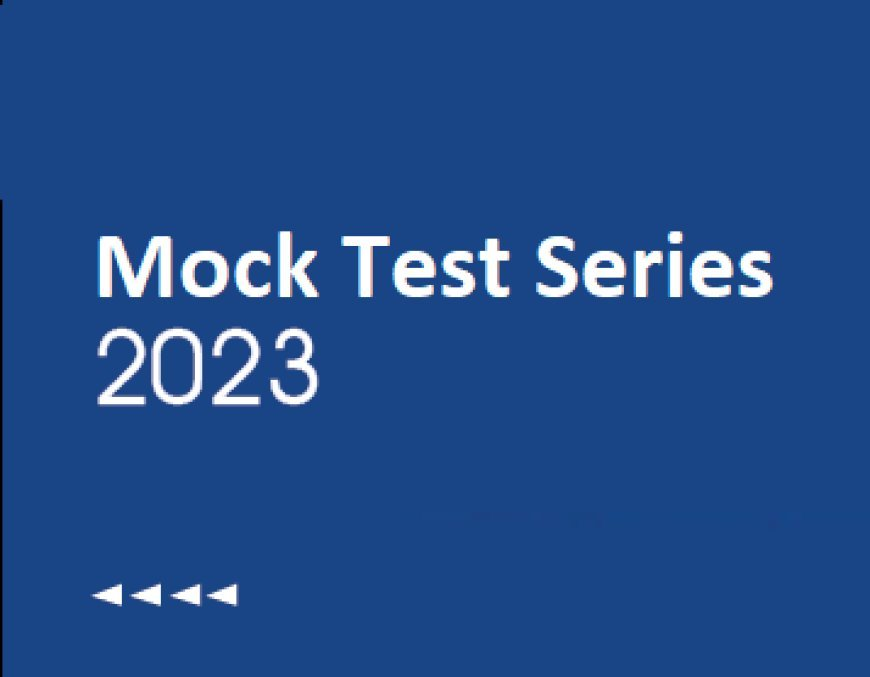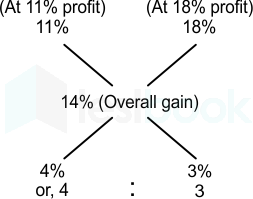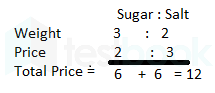# AAO Exam-CT 8: Quant (Profit and Loss)

## AAO Exam-CT 8- Quant (Profit and Loss)

0  4691. If a product is sold at a 5% discount, the selling price is Rs. 266. When selling it on Marked Price, if the profit percentage is 12% then find the Cost price of the product.

• Rs. 210
• Rs. 220
• Rs. 230
• Rs. 240
• Rs. 250

Given:

If a product is sold at 5% discount, the selling price is Rs. 266.

Formula used:

S.P = M.P × (100 – D)/100

Where,

S.P → Selling price

M.P → Marked price

D → Discount%

Calculations:

Suppose the marked price is Rs. M

S.P = M × (100 – 5)/100 = 0.95M

⇒ 0.95M = 266

⇒ M = Rs. 280

Marked price = Rs. 280

Now ,

112% of Cost Price = 280

Cost Price = 280/112 × 100 = Rs. 250

2. Prakash buys an almirah at Rs. 8000 and spends Rs. 2000 on its repair. He sells it to Rakesh at Rs. 12,500. Find the profit percentage earned by Prakash.
• 12.5%
• 15%
• 25%
• 30%
• 10%

Given:

Purchase price of the almirah is Rs. 8000

Cost of its repair is Rs. 2000

And the selling price of the almirah is Rs. 12,500.

Formula used:

Profit = S.P – C.P

And Profit% = (Profit/C.P) × 100

Where, C.P = Cost Price and S.P = Selling

Calculation:

Total cost price (C.P) of the almirah = Purchase price + Repair cost

⇒ Rs. 8000 + Rs. 2000

⇒ Rs. 10,000

Now, Profit = S.P – C.P

⇒ Rs. 12,500 – Rs. 10,000

⇒ Rs. 2500

So, Profit% = (Profit/C.P) × 100

⇒ (2500/10,000) × 100

⇒ 25%

∴ 25% profit is earned by Prakash.

3. A merchant sells 980 kg of fruits in two parts. One at 11% profit and other at 18% profit. He gains 14% on overall selling. Find how much quantity he sold at 18% profit?
• 490 kg
• 430 kg
• 410 kg
• 420 kg
• None of these

Given:

Total fruits sold = 980 kg.

Profit percentage is 11% and 18% respectively.

Overall gain = 14%

Concept used:

We will be using the concept of alligation.

Calculation:

Using allegation,Or 4 : 3

Total units,

7 units = 980 kg

1 units = 140 kg

3 unit = 420 kg

4. X and Y started a business by investing Rs. 3000 and Rs. 4500 respectively. After 5 months, X invested Rs. 2000 more while Y withdrew Rs. 1000, find the ratio of profit of Y and X respectively after an year.
• 50 : 47
• 47 : 50
• 25 : 47
• 50 : 43
• 50 : 53

Given:

Investment of X and Y = Rs. 3000 and Rs. 4500 respectively

After 5 months, X invested Rs. 2000 more while Y withdrew Rs. 1000

Formula Used:

Profit = Investment × Time

Calculations:

Investment of X = Rs. 3000

Investment of Y = Rs. 4500

After 5 months, X invested Rs. 2000 more while Y withdrew Rs. 1000

⇒ Total investment of X after 5 months = Rs. (3000 + 2000) = Rs. 5000

Total investment of Y after 5 months = Rs. (4500 - 1000) = Rs. 3500

After 1 year,

Total profit of X = 3000 × 5 + 5000 × (12 - 5)

Total profit of Y = 4500 × 5 + 3500 × (12 - 5)

⇒ Ratio of profits of Y and X = (4500 × 5 + 3500 × 7) : ( 3000 × 5 + 5000 × 7)

⇒ Ratio of profits of Y and X = 47 : 50

∴ The ratio of profit of Y and X respectively after an year is 47 : 50.

5. P and Q entered into a partnership with their capitals in the ratio 5 : 2. At the end of 10 months, P withdraw his capital. If they receives the profit in the ratio 5 : 1, Find how long Q capital was used?
• 3 months
• 2 months
• 7 months
• 5 months
• 9 months

Given:

Ratio of P and Q capital = 5 : 2.

Formula used:

Share of profit = amount invested × time period

Calculation:

Ratio of share of profit = P : Q

(5 × 10) : (2 × t) = 5 : 1

t = 5 months

∴ Q  capital was used for 5 months.
6. A buys two books. The cost price of two books is Rs.700 each. One books sells at 10% profit and other 15% profit. Find the total profit percentage.
• 12%
• 15%
• 14.5%
• 12.5%
• 13.5%

Given:

The Cost Price of the two books = Rs.700

Profit on selling one book = 10%

Profit on selling another book = 15%

Formula Used:

If an article is sold at a Profit of x%, the Selling Price becomes:

SP = [(100 + x)/100] × CP

Calculation:

The Cost Price of the two books = Rs.700

Selling price of first book = (100 + 10)/100 × 700 = Rs.770

Selling price of second book = (100 + 15)/100 × 700 = Rs.805

Total Cost price = 700 + 700 = Rs.1400

Total Selling price = 770 + 805 = Rs.1575

Total profit = 1575 - 1400 = Rs.175

Profit% = 175/1400 × 100 = 12.5%

∴ The total profit percentage is 12.5%.The total percentage when CP of two object is same = The average of profit%

⇒ (10 + 15)/2 = 25/2 = 12.5

∴ The total profit percentage is 12.5%.Mistake Points

This is possible on when CP is same and for two articles.

Don't use the formula of successive percentage(net percentage)

7. Company marks a article 25% above the cost price and sold it at Rs 6480 by giving two successive discounts of 20% and 10% each. Find cost price of product and loss/ profit %.
• Rs 8500, loss of 20%
• Rs 7200, Loss of 10%
• Rs 6400, Loss of 10%
• Rs 7200, Profit of 10%
• Rs 6400, Profit of 10%

Given:

Mark up = 25%

S.P. = Rs 6480

Successive discounts = 20% and 10% each.

Formula used:

Marked price – (discount × marked price/ 100) = Selling price

Cost price = Marked price/ (1 + (r/ 100))

Where, r = marked up %

Loss % = ((C.P. – S.P.) / C.P.) × 100

Effective discount% = a + b – ((a × b)/ 100)

Where a, b are successive discounts in %

Calculation:

Effective discount % = 20 + 10 – (20 × 10/ 100)

⇒ Effective discount = 30 – 2

⇒ Effective discount = 28%

⇒ Marked price of article = 6480/ (1 – 0.28)

⇒ M.P. = 6480/ 0.72

⇒ M.P. = 9000

C.P. of article = M.P. / (1 + 0.25)

⇒ C.P. of article = 9000/ 1.25

⇒ C.P. of article = Rs 7200

Loss % = ((7200 – 6480) / 7200) × 100

⇒ Loss% = 720 × 100/ 7200

⇒ Loss% = 10%

∴ Cost price of article is Rs 7200 and loss % is 10%

8. Ram bought some sugar and salt for Rs. 480. The ratio of weight of sugar and salt is 3 : 2. The ratio of price of sugar and salt is 2 : 3. Find the price of sugar.
• Rs. 240
• Rs. 220
• Rs. 330
• Rs. 200
• Rs. 400

Given:

Price of sugar and salt = Rs. 480

Ratio of weight of sugar and salt is 3 : 2

Ratio of price of sugar and salt is 2 : 3.

Calculation:⇒ 12 units = Rs.480

⇒ 1 Unit = 480/12 = Rs.40

∴ Price of sugar = 40 × 6 = Rs. 240

9. Two friends Rahul and Roshan started a business by investing Rs. 24000 and Rs. 32000 respectively. They decide that 50% of the profit should be divided equally among them and the rest of the amount is divided into their investment ratio. If Roshan got Rs. 4000 more than Rahul, what is the total profit?

• 50000
• 53520
• 56000
• 54050
• None of these

Given:

Invest amount of Rahul  = Rs. 24000

Invest amount of Roshan  = Rs. 32000

Equally divided amount of profit = 50%

Divided amount of profit into their investment ratio = 50%

Calculation:

Let, the total profit = 100x

Investment ratio b/w Rahul and Roshan = 24000 : 32000 = 3 : 4

According to the question,

⇒  [(4/7) ─ (3/7)] × 50x = 4000

⇒ [(1/7)] × 50x = 4000

⇒ 100x = 56000

∴ Total profit = Rs. 56000

10. A man buys cotton at Rs. 2.5/kg with a minimum purchase of 20 kg. If he pays Rs. 80 for some cotton, by how much kilograms did his purchase exceeds the minimum purchase?

• 15 kg
• 12 kg
• 10 kg
• 17 kg
• 20 kg

Formula Used:

Total Price = Rate × weight purchased

Calculation:

Assume he buys x kilograms of cotton at Rs.2.5/kg

⇒ 2.5x = 80

⇒ x = 80/2.5 = 32

∴ The amount that exceeds the minimum purchase = 32 – 20 = 12 kg

### AAO Exam-CT 9: Quant (Time and Work)